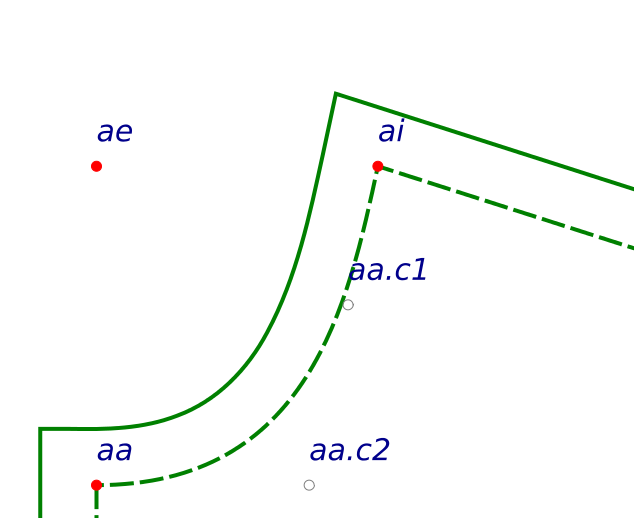# Basics: Variables in TMTPThe basic data object in TMTP is the point.

A point is a python object with these three attributes:
x       : the x coordinate of the point
y       : the y coordinate of the point
name: the name of the point

The names are very simplified, because there can be over 100 points in a complex pattern piece. Points are named using it’s pattern piece letter. The first point to be drawn on  pattern piece D should be named ‘da‘.

There are two types of drawn points in TMTP:
pattern points     : solid red circles, created with rPointP() or rPoint() functions.
control points     : gray outlined circles, created with cPointP() or cPoint() functions.
Pattern points mark normal pattern points.
Control points mark the handles of pattern curves.

Here is TMTP code to create points da at the origin and db at 3 inches right and 4 inches down from da on pattern piece D:
da = rPoint(D, ‘da’, 0.0, 0.0)
db = rPoint(D, ‘db’, da.x + 3.0*IN, da.y + 4*IN)

To calculate control points for a curve from da to db:
db.c1 = cPointP(D, ‘db.c1’, <point at angle and length from da>)
db.c2 = cPointP(D, ‘db.c2’, <point at angle and length from db>)
more on how to calculate control points in another tutorial
–  they’re usually created at an angle from one of the two endpoints.

IN converts inches to pixels, and CM converts centimeters to pixels. TMTP uses Inkscape’s base measurement ratio, 90 pixels per inch. Look in the tmtp/standalone/tmtpl/constants.py file to see all the TMTP global variables.

Python Programming Notes:
The numbers used with IN and CM can be integers or have decimal values. The result will be the same. When using fractions, ratios, or dividing, as in (5/8.0)*IN, the divisor *must* have a decimal or python may round down to the nearest integer.

Some points do not need to create an SVG circle.  You might need to create a point to help calculate a pattern or control point:
temp_pnt = Pnt(db.x, da.y)
The Pnt() function creates a python object of the Pnt base class. Pattern and control points are created with the Point() base class which has attributes and methods to enable the point to be drawn to the SVG canvas, transformed, etc.

Here’s an example of some of pattern point da’s attributes, on pattern piece D, with coordinates (15, 20):
da.x   = 15.0
da.y   = 20.0
da.name = ‘da’
da.id = ‘D.da’
da.coords = ‘15.0, 20.0’